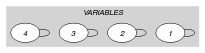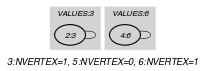## 5.298. open_global_cardinality

Origin
Constraint

$\mathrm{𝚘𝚙𝚎𝚗}_\mathrm{𝚐𝚕𝚘𝚋𝚊𝚕}_\mathrm{𝚌𝚊𝚛𝚍𝚒𝚗𝚊𝚕𝚒𝚝𝚢}\left(𝚂,\mathrm{𝚅𝙰𝚁𝙸𝙰𝙱𝙻𝙴𝚂},\mathrm{𝚅𝙰𝙻𝚄𝙴𝚂}\right)$

Synonyms

$\mathrm{𝚘𝚙𝚎𝚗}_\mathrm{𝚐𝚌𝚌}$, $\mathrm{𝚘𝚐𝚌𝚌}$.

Arguments
 $𝚂$ $\mathrm{𝚜𝚟𝚊𝚛}$ $\mathrm{𝚅𝙰𝚁𝙸𝙰𝙱𝙻𝙴𝚂}$ $\mathrm{𝚌𝚘𝚕𝚕𝚎𝚌𝚝𝚒𝚘𝚗}\left(\mathrm{𝚟𝚊𝚛}-\mathrm{𝚍𝚟𝚊𝚛}\right)$ $\mathrm{𝚅𝙰𝙻𝚄𝙴𝚂}$ $\mathrm{𝚌𝚘𝚕𝚕𝚎𝚌𝚝𝚒𝚘𝚗}\left(\mathrm{𝚟𝚊𝚕}-\mathrm{𝚒𝚗𝚝},\mathrm{𝚗𝚘𝚌𝚌𝚞𝚛𝚛𝚎𝚗𝚌𝚎}-\mathrm{𝚍𝚟𝚊𝚛}\right)$
Restrictions
 $𝚂\ge 1$ $𝚂\le |\mathrm{𝚅𝙰𝚁𝙸𝙰𝙱𝙻𝙴𝚂}|$ $\mathrm{𝚛𝚎𝚚𝚞𝚒𝚛𝚎𝚍}$$\left(\mathrm{𝚅𝙰𝚁𝙸𝙰𝙱𝙻𝙴𝚂},\mathrm{𝚟𝚊𝚛}\right)$ $\mathrm{𝚛𝚎𝚚𝚞𝚒𝚛𝚎𝚍}$$\left(\mathrm{𝚅𝙰𝙻𝚄𝙴𝚂},\left[\mathrm{𝚟𝚊𝚕},\mathrm{𝚗𝚘𝚌𝚌𝚞𝚛𝚛𝚎𝚗𝚌𝚎}\right]\right)$ $\mathrm{𝚍𝚒𝚜𝚝𝚒𝚗𝚌𝚝}$$\left(\mathrm{𝚅𝙰𝙻𝚄𝙴𝚂},\mathrm{𝚟𝚊𝚕}\right)$ $\mathrm{𝚅𝙰𝙻𝚄𝙴𝚂}.\mathrm{𝚗𝚘𝚌𝚌𝚞𝚛𝚛𝚎𝚗𝚌𝚎}\ge 0$ $\mathrm{𝚅𝙰𝙻𝚄𝙴𝚂}.\mathrm{𝚗𝚘𝚌𝚌𝚞𝚛𝚛𝚎𝚗𝚌𝚎}\le |\mathrm{𝚅𝙰𝚁𝙸𝙰𝙱𝙻𝙴𝚂}|$
Purpose

Each value $\mathrm{𝚅𝙰𝙻𝚄𝙴𝚂}\left[i\right].\mathrm{𝚟𝚊𝚕}$ $\left(1\le i\le |\mathrm{𝚅𝙰𝙻𝚄𝙴𝚂}|\right)$ should be taken by exactly $\mathrm{𝚅𝙰𝙻𝚄𝙴𝚂}\left[i\right].\mathrm{𝚗𝚘𝚌𝚌𝚞𝚛𝚛𝚎𝚗𝚌𝚎}$ variables of the $\mathrm{𝚅𝙰𝚁𝙸𝙰𝙱𝙻𝙴𝚂}$ collection for which the corresponding position belongs to the set $𝚂$. Positions are numbered from 1.

Example
$\left(\begin{array}{c}\left\{2,3,4\right\},\hfill \\ 〈3,3,8,6〉,\hfill \\ 〈\begin{array}{cc}\mathrm{𝚟𝚊𝚕}-3\hfill & \mathrm{𝚗𝚘𝚌𝚌𝚞𝚛𝚛𝚎𝚗𝚌𝚎}-1,\hfill \\ \mathrm{𝚟𝚊𝚕}-5\hfill & \mathrm{𝚗𝚘𝚌𝚌𝚞𝚛𝚛𝚎𝚗𝚌𝚎}-0,\hfill \\ \mathrm{𝚟𝚊𝚕}-6\hfill & \mathrm{𝚗𝚘𝚌𝚌𝚞𝚛𝚛𝚎𝚗𝚌𝚎}-1\hfill \end{array}〉\hfill \end{array}\right)$

The $\mathrm{𝚘𝚙𝚎𝚗}_\mathrm{𝚐𝚕𝚘𝚋𝚊𝚕}_\mathrm{𝚌𝚊𝚛𝚍𝚒𝚗𝚊𝚕𝚒𝚝𝚢}$ constraint holds since:

• Values 3, 5 and 6 respectively occur 1, 0 and 1 times within the collection $〈3,3,8,6〉$ (the first item 3 of $〈3,3,8,6〉$ is ignored since value 1 does not belong to the first argument $S=\left\{2,3,4\right\}$ of the $\mathrm{𝚘𝚙𝚎𝚗}_\mathrm{𝚐𝚕𝚘𝚋𝚊𝚕}_\mathrm{𝚌𝚊𝚛𝚍𝚒𝚗𝚊𝚕𝚒𝚝𝚢}$ constraint).

• No constraint was specified for value 8.

Typical
 $|\mathrm{𝚅𝙰𝚁𝙸𝙰𝙱𝙻𝙴𝚂}|>1$ $\mathrm{𝚛𝚊𝚗𝚐𝚎}$$\left(\mathrm{𝚅𝙰𝚁𝙸𝙰𝙱𝙻𝙴𝚂}.\mathrm{𝚟𝚊𝚛}\right)>1$ $|\mathrm{𝚅𝙰𝙻𝚄𝙴𝚂}|>1$ $\mathrm{𝚛𝚊𝚗𝚐𝚎}$$\left(\mathrm{𝚅𝙰𝙻𝚄𝙴𝚂}.\mathrm{𝚗𝚘𝚌𝚌𝚞𝚛𝚛𝚎𝚗𝚌𝚎}\right)>1$ $|\mathrm{𝚅𝙰𝚁𝙸𝙰𝙱𝙻𝙴𝚂}|>|\mathrm{𝚅𝙰𝙻𝚄𝙴𝚂}|$
Symmetries
• Items of $\mathrm{𝚅𝙰𝙻𝚄𝙴𝚂}$ are permutable.

• An occurrence of a value of $\mathrm{𝚅𝙰𝚁𝙸𝙰𝙱𝙻𝙴𝚂}.\mathrm{𝚟𝚊𝚛}$ that does not belong to $\mathrm{𝚅𝙰𝙻𝚄𝙴𝚂}.\mathrm{𝚟𝚊𝚕}$ can be replaced by any other value that also does not belong to $\mathrm{𝚅𝙰𝙻𝚄𝙴𝚂}.\mathrm{𝚟𝚊𝚕}$.

Usage

In their article [HoeveRegin06], W.-J. van Hoeve and J.-C. Régin motivate the $\mathrm{𝚘𝚙𝚎𝚗}_\mathrm{𝚐𝚕𝚘𝚋𝚊𝚕}_\mathrm{𝚌𝚊𝚛𝚍𝚒𝚗𝚊𝚕𝚒𝚝𝚢}$ constraint by the following scheduling problem. Consider a set of activities (where each activity has a fixed duration 1 and a start variable) that can be processed on two factory lines such that all the activities that will be processed on a given line must be pairwise distinct. This can be modelled by using one $\mathrm{𝚘𝚙𝚎𝚗}_\mathrm{𝚐𝚕𝚘𝚋𝚊𝚕}_\mathrm{𝚌𝚊𝚛𝚍𝚒𝚗𝚊𝚕𝚒𝚝𝚢}$ constraint for each line, involving all the start variables as well as a set variable whose final value specifies the set of activities assigned to that specific factory line.

Note that this can also be directly modelled by a single $\mathrm{𝚍𝚒𝚏𝚏𝚗}$ constraint. This is done by introducing an assignment variable for each activity. The initial domain of each assignment variable consists of two values that respectively correspond to the two factory lines.

Remark

In their article [HoeveRegin06], W.-J. van Hoeve and J.-C. Régin consider the case where we have no counter variables for the values, but rather some lower and upper bounds (i.e., in fact the $\mathrm{𝚘𝚙𝚎𝚗}_\mathrm{𝚐𝚕𝚘𝚋𝚊𝚕}_\mathrm{𝚌𝚊𝚛𝚍𝚒𝚗𝚊𝚕𝚒𝚝𝚢}_\mathrm{𝚕𝚘𝚠}_\mathrm{𝚞𝚙}$ constraint).

Algorithm

A slight adaptation of the flow model that handles the original $\mathrm{𝚐𝚕𝚘𝚋𝚊𝚕}_\mathrm{𝚌𝚊𝚛𝚍𝚒𝚗𝚊𝚕𝚒𝚝𝚢}$ constraint [Regin96] is described in [HoeveRegin06].

specialisation: $\mathrm{𝚘𝚙𝚎𝚗}_\mathrm{𝚊𝚕𝚕𝚍𝚒𝚏𝚏𝚎𝚛𝚎𝚗𝚝}$ (each active valueAn active value corresponds to a value occuring at a position mentionned in the set $𝚂$. should occur at most once), $\mathrm{𝚘𝚙𝚎𝚗}_\mathrm{𝚐𝚕𝚘𝚋𝚊𝚕}_\mathrm{𝚌𝚊𝚛𝚍𝚒𝚗𝚊𝚕𝚒𝚝𝚢}_\mathrm{𝚕𝚘𝚠}_\mathrm{𝚞𝚙}$ ($\mathrm{𝚟𝚊𝚛𝚒𝚊𝚋𝚕𝚎}$ replaced by $\mathrm{𝚏𝚒𝚡𝚎𝚍}$ $\mathrm{𝚒𝚗𝚝𝚎𝚛𝚟𝚊𝚕}$).

Keywords

For all items of $\mathrm{𝚅𝙰𝙻𝚄𝙴𝚂}$:

Arc input(s)

$\mathrm{𝚅𝙰𝚁𝙸𝙰𝙱𝙻𝙴𝚂}$

Arc generator
$\mathrm{𝑆𝐸𝐿𝐹}$$↦\mathrm{𝚌𝚘𝚕𝚕𝚎𝚌𝚝𝚒𝚘𝚗}\left(\mathrm{𝚟𝚊𝚛𝚒𝚊𝚋𝚕𝚎𝚜}\right)$

Arc arity
Arc constraint(s)
 $•\mathrm{𝚟𝚊𝚛𝚒𝚊𝚋𝚕𝚎𝚜}.\mathrm{𝚟𝚊𝚛}=\mathrm{𝚅𝙰𝙻𝚄𝙴𝚂}.\mathrm{𝚟𝚊𝚕}$ $•$$\mathrm{𝚒𝚗}_\mathrm{𝚜𝚎𝚝}$$\left(\mathrm{𝚟𝚊𝚛𝚒𝚊𝚋𝚕𝚎𝚜}.\mathrm{𝚔𝚎𝚢},𝚂\right)$
Graph property(ies)
$\mathrm{𝐍𝐕𝐄𝐑𝐓𝐄𝐗}$$=\mathrm{𝚅𝙰𝙻𝚄𝙴𝚂}.\mathrm{𝚗𝚘𝚌𝚌𝚞𝚛𝚛𝚎𝚗𝚌𝚎}$

Graph model

Since we want to express one unary constraint for each value we use the “For all items of $\mathrm{𝚅𝙰𝙻𝚄𝙴𝚂}$” iterator. The only difference with the graph model of the $\mathrm{𝚐𝚕𝚘𝚋𝚊𝚕}_\mathrm{𝚌𝚊𝚛𝚍𝚒𝚗𝚊𝚕𝚒𝚝𝚢}$ constraint is the arc constraint where we also specify that the position of the considered variable should belong to the first argument $𝚂$.

Part (A) of Figure 5.298.1 shows the initial graphs associated with each value 3, 5 and 6 of the $\mathrm{𝚅𝙰𝙻𝚄𝙴𝚂}$ collection of the Example slot. Part (B) of Figure 5.298.1 shows the two corresponding final graphs respectively associated with values 3 and 6 that are both assigned to those variables of the $\mathrm{𝚅𝙰𝚁𝙸𝙰𝙱𝙻𝙴𝚂}$ collection for which the index belongs to $𝚂$ (since value 5 is not assigned to any variable of the $\mathrm{𝚅𝙰𝚁𝙸𝙰𝙱𝙻𝙴𝚂}$ collection the final graph associated with value 5 is empty). Since we use the $\mathrm{𝐍𝐕𝐄𝐑𝐓𝐄𝐗}$ graph property, the vertices of the final graphs are stressed in bold.

##### Figure 5.298.1. Initial and final graph of the $\mathrm{𝚘𝚙𝚎𝚗}_\mathrm{𝚐𝚕𝚘𝚋𝚊𝚕}_\mathrm{𝚌𝚊𝚛𝚍𝚒𝚗𝚊𝚕𝚒𝚝𝚢}$ constraint(a) (b)